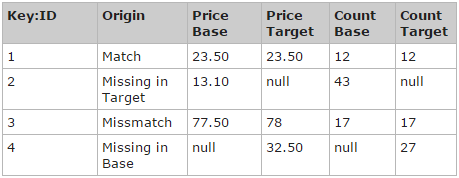“Summarise data in tables.”### Get Summary Statistics

Aggregates table results in columns applying specific conditions to each column. It can add and count values and it can display average results and maximum and minimum values. The allowed functions are SUM(), AVG(), COUNT(), MAX() and MIN().br />

#### Input parameters:

Summarise Columns - Name of the columns to aggregate. It needs to include the functions used to calculate the results and the name of the column to apply the function in E.g “SUM(ColumnName)”
Group By Columns - Name of the column(s) to group by.

###### Example Data

For the following operations let’s assume you have the following data: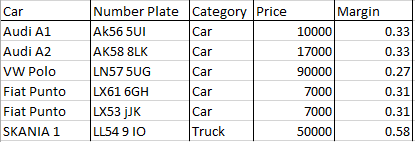###### Example 1:

In our case, if you wanted to know the total price of all produces in various categories you would just specify:

Aggregate Columns: SUM(Price)
Group By Columns: Product Category

###### Example 2:

If you wanted to know the Average Margin across all the products, you would specify:

Aggregate Columns: AVG(PRICE)
Group By Columns: Product Category

### Create Pivot table

Generate a table with data grouped in Row and Column fields.

#### Input parameters:

Row Fields - Data field(s) to group by horizontally
Column Fields - Data field(s) to group by vertically
Data Fields - Data field(s) to calculate and/or display across the horizontal and vertical dimensions. You can use these functions to calculate the resulting values: SUM(), AVG(), COUNT(), MAX() and MIN().

##### Examples:

A simple reconciliation of two tables with three columns (ID, Price, and Count), might produce a denormalized result set like this: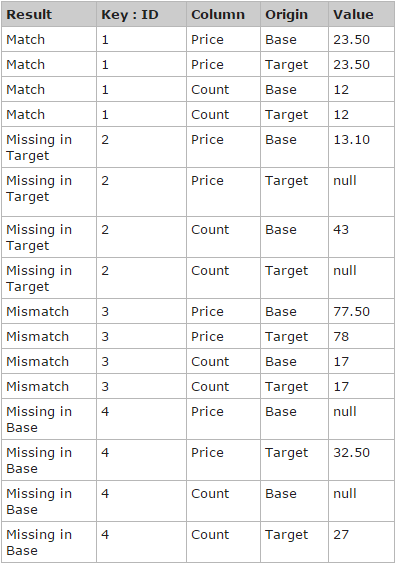To group results by column, you can pivot this output with KEY:ID+COLUMN+RESULT against ORIGIN to produce this: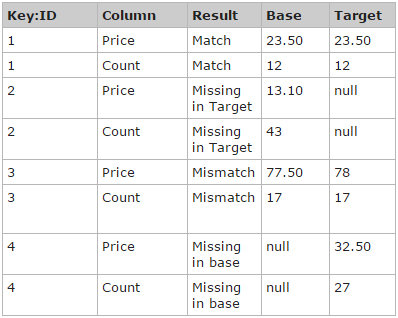To group results by origin, you can pivot this output with KEY:ID+ORIGIN+RESULT against COLUMN to produce this: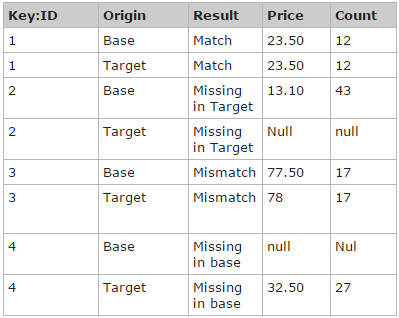If you just want one result per row, you can pivot the output on KEY:ID+RESULT against COLUMN+ORIGIN, producing